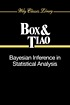# Classify

## Summary

Title:
Bayesian inference in statistical analysis
Author:
Box, George E. P; Tiao, George C., 1933-; WISCONSIN UNIV MADISON Dept. of STATISTICS
Formats:

Editions:
47
Total Holdings:
1278
OCLC Work Id:
416709
http://classify.oclc.org/classify2/ClassifyDemo?owi=416709
DDC: Class Number Holdings Links
Most Frequent 519.54 1209 Web Dewey
Edition: 22 519.54 10
LCC: Class Number Holdings Links
Most Frequent QA276 1154 ClassWeb## FAST Subject Headings

Heading Usage Count FAST ID
Mathematical statistics 1167 1012127
Mathematics 16 1012163## Editions

Displaying 1 to 25 of 47
Title and AuthorLanguageFormatHoldings TagClass NumberBayesian inference in statistical analysisby Box, George E. Peng 617050QA276
082519.54Bayesian inference in statistical analysisby Box, George E. Peng 258082519.54

(DDC 20)

050QA276Bayesian inference in statistical analysisby Box, George E. Peng 238082519.54

(DDC 20)

050QA276Bayesian inference in statistical analysisby Box, George E. P.eng 20082519.54Bayesian inference in statistical analysisby Box, George E. Peng 16082519.542Bayesian inference in statistical analysisby Box, George E. Peng 14082519.54

(DDC 20)

050QA276Bayesian inference in statistical analysisby Box, George E. Peng 11082519.92Bayesian inference in statistical analysisby Box, George E. Peng 10050QA276
082519.54

(DDC 22)Bayesian inference in statistical analysisby Box, George Edward Pelhameng 10082519.54Bayesian inference in statistical analysiseng 10082519.54Bayesian inference in statistical analysisby Box, George Edward Pelhameng 9Bayesian inference in statistical analysisby Box, George E. Peng 9050QA276
082519.54Bayesian inference in statistical analysisby Box, George E. Peng 6082519.54

(DDC 20)Bayesian inference in statistical analysisby Box, George E. Peng 5050QA279.5Bayesian inference in statistical analysisby Box, George E. Peng 3Bayesian inference in statistical analysisby Box, George E. P. (George Edward Pelham)eng 3082519.542Bayesian inference in statistical analysisby Box, George E. Peng 3050QA276
082519.54
082519.542Bayesian Inference in Statistical Analysisby Box, George E. Peng 3050QA276
082510Bayesian Inference in Statistical Analysiseng 2082519.54

(DDC 20)Bayesian inference in statistical analysisby Box, George Eeng 2082519.54

(DDC 18)

050QA279.5Bayesian inference in statistical analysisby Box, George E. P. (George Edward Pelham)eng 2082519.54

(DDC 20)Bayesian Inference in Statistical Analysisby Box, George E. Peng 2082519.54Bayesian inference in statistical analysisby Box, George E. Punk 1Bayesian inference in statistical analysisby Box, George E. Peng 1Bayesian inference in statistical analysisby Box, George E. Peng 1
Displaying 1 to 25 of 47
.